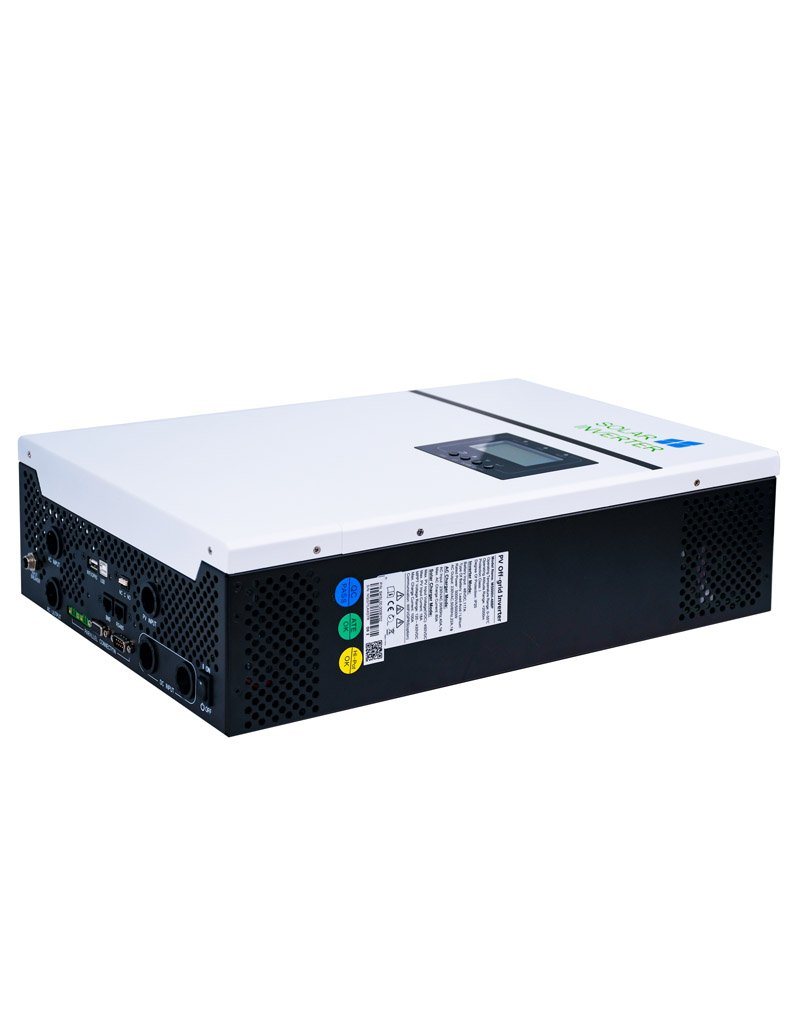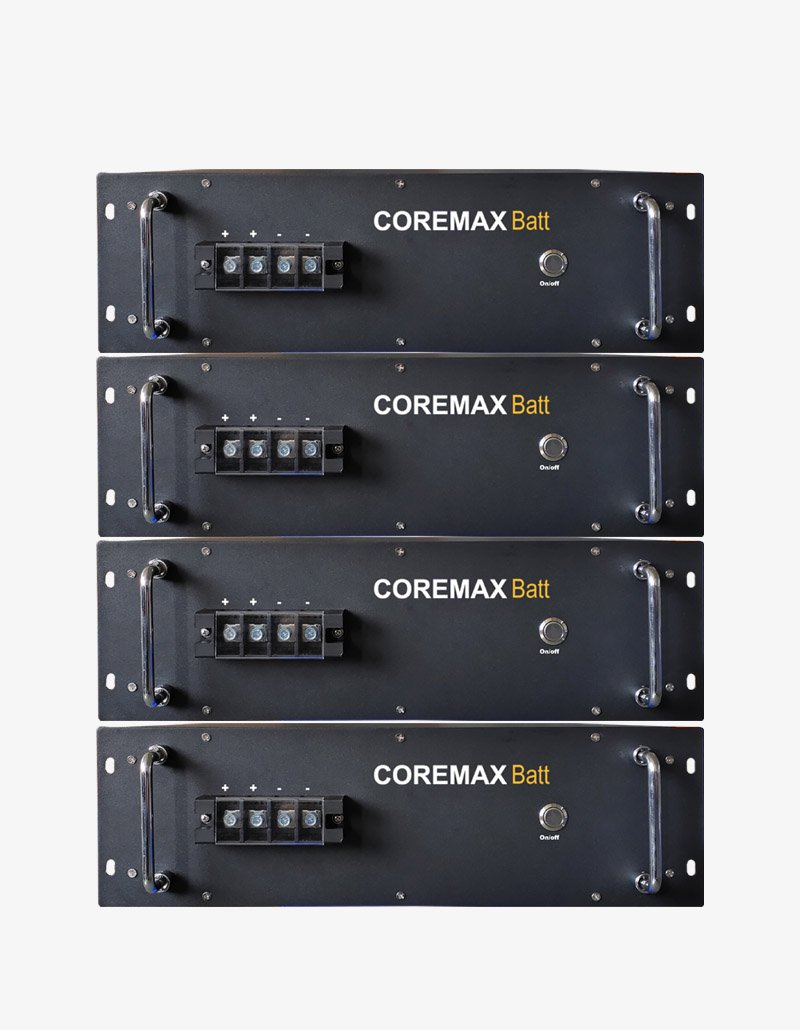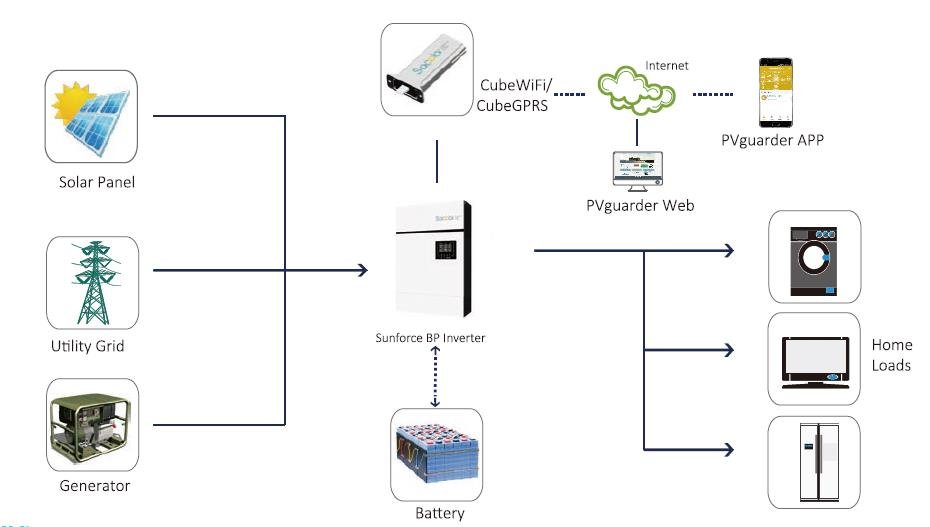Solar energy storage system

# How many lithium batteries required for a 5000w solar inverter?

5000w solar inverter is the most popular watt for home solar energy storage system. When you build the solar system, a lithium battery storage system is normally required no matter for off grid or on grid system. Mostly people will think about a question, how many batteries I should have for my 5kw inverter. What should I considering when I plan for the battery system. Here let's talk about this question and hopefully can brings you some valuable ideal or thought.## What is the jobs for a solar inverter

Inverters are used to change direct current (DC) to alternating current (AC), and the power inverters can power a household with standard 120V/240v alternating current when most home appliances for home use are rated at 42.5 amps. That is to say, besides the number of batteries needed to power the 5000-watt inverter, you also need to take battery capacity, voltage input of the inverter, and the time duration you use the inverter into consideration.Ideally speaking, the capacity of the battery is discharged 100% but the fact is not. If you choose lithium battery instead of lead-acid battery, the DOD can be as high as 80% instead of 50%, which means the inverter gets 80% of the lithium battery power. When considering the inverter voltage input, you need to know the typical and surge power rating as well as the average power.

The first step is to calculate how much power is needed by summing up the power consumption of the home appliances. Besides the usual power need, the inverters also need to supply the surge power which usually last for a short time ranging from a few seconds to at most 15 minutes. For example, the following loads are supposed to be caried by the inverter.

• Five lighting load, 500W in total
• Two fans, which is 140W altogether
• One refrigerator with the surge power at 240W
• Then you need to check whether the inverter input voltage matches the batteries, that is, you will need two of 24V lithium battery or one 48V lithium batteries instead if the input voltage of the inverter is 48V.

The next essential question is to consider how long you want the inverter to last as the backup power source. By answering this question, you know the required watt hours and battery capacity. Take the 48V 5000W rating as an example for the lithium ion inverter, and you want the inverter to run for at least 5 hours. Load factor is the efficiency of electrical energy usage, calculated by dividing the average load into the peak load in a specified time period. Assuming the load factor is as high as 80%, the volt amperes is equal to 5000W/48v/80%=130 amperes which is the smallest capacity provided by the lithium battery. As a result, 200ah lithium battery is required when other capacity rating more than 130ah is not common.

The last step is to calculate the storage capacity of the lithium battery. As calculated above, the required battery capacity is 200ah which can run the 5000W inverter for home power backup system. Since battery power (Wh)= battery capacity (AH) X battery voltage (V) X number of batteries = 200X48X1or 200X24X2=9600Wh, the backup time = battery power (Wh) / connected load (W) =9600 / (500+140+240) =10.91 hours.

All in All. There are many factors you need considering for numbers of batteries for 5000 watt inverter. If you still not clear, why not just simple contact us. We will help you find out and provide most professional proposal.